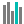#CONFIDENCE.T (PQL - xl)

This function returns the confidence interval for a population mean

• Library: PQL \ Spreadsheet \ Statistical
• Compatibility: Any content (regardless of data source) in the Tabulate spreadsheet module

#### Syntax

CONFIDENCE.T(alpha, standard dev, size)

##### Function Arguments
 Name Description Type Optional alpha Alpha, significance level used in calculating confidence level, where the confidence level is 100 times (1-alpha)% Number standard dev Population standard deviation for the range Number size Number representing the size of the sample; if not an integer, the number is truncated Number